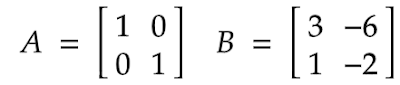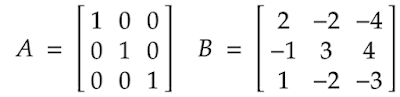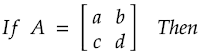# Idempotent matrix – Definition, Examples and its properties

0
531

## What is an Idempotent matrix?

Definition: Mathematically we can define Idempotent matrix as: a square matrix [A] will be called Idempotent matrix if and only if it satisfies the condition  A2 = A. Where A is n x n square matrix.

In other words, an Idempotent matrix is a square matrix which when multiplied by itself, gives result as same square matrix.

Also if square of any matrix gives same matrix ( i.e, A2 = A )  then that matrix will be Idempotent matrix.

Here if we observe the definition  A2A, i.e, A = square of (A). It means we can say that the Idempotent matrix [A] is always the square of same matrix [A].

## Examples of Idempotent matrix

### Example of 2 x 2 Idempotent matrix### Example of 3 x 3 Idempotent matrix## Conditions of Idempotent matrix

The necessary conditions for any 2 x 2 square matrix to be an Idempotent matrix is that either it should be diagonal matrix of order 2 x 2, or its trace value should be equal to 1.

## Properties of Idempotent matrix

These are important properties of Idempotent matrix.

1. If any Idempotent matrix is identity matrix [I], then it will be non-singular matrix.
2. When any Idempotent matrix [A] is subtracted from identity matrix [I], then the resultant matrix [I-A] will also be an idempotent matrix.
3. If a non-identity matrix is an idempotent matrix then its number of independent rows and columns will always be less than the number of total rows and columns of that Idempotent matrix.
4. If a matrix [A] is an idempotent matrix, then for all positive integer values of variable n‘, the result An = A will always true.
5. The Eigen values of any Idempotent matrix will always be either or 1. That means an idempotent matrix is always diagonalizable.
6. The trace of an idempotent matrix will be equal to the rank of that Idempotent matrix, hence trace will always be an integer value.
7. For any 2 x 2 idempotent matrix [A].• a = a2 + bc
• b = ab + bc, implying that  b(1 – a – d) = 0, so either b = 0, or d = (1 – a)
• c = ac + dc, implying that  c(1 – a – d) = 0, so either c = 0, or d = (1 – a)
• d = d2 + bc

## Application of Idempotent matrix

One of the very important applications of Idempotent matrix is that it is very easy and useful for solving [ M ] matrix and Hat matrix during regression analysis and econometrics.

The idempotency of  [ M ]  matrix plays very important role in other calculations of  regression analysis and econometrics.